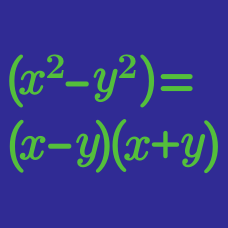Algebra

# Factoring Polynomials by Grouping

Find the sum of all real solutions of the equation $x^3+x^2 + x=8x^2+8 x + 8.$

The polynomial in two variables

$f(x,y) = xy + 3y - 4x -12$

can be expressed as the product of two linear factors with integer coefficients, namely

$f(x,y) = (ax + by + c) ( dx + ey + f).$

Evaluate $|a+b+c| + |d+e+f|$.

If $a, b, c$ and $d$ are positive numbers and $5x^3-45xy^2+4x^2-36y^2=(ax+b)(x+cy)(x-dy),$ what is the value of $a+b+c+d$?

If $a, b, c,$ and $d$ are positive numbers such that \begin{aligned} & (x^2-5x)^2-18x^2+90x-144 \\ &= (x-a)(x-b)(x-c)(x+d), \end{aligned} what is the value of $a+b+c+d$?

If $a$ and $b$ are positive numbers such that $x^3+5x^2y-9x-45y=(x+a)(x-b)(x+cy),$ what is the value of $a+b+c$?

×